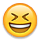True power of a 24 volt battery bank relative to a 12 volt bank.

Registered Users Posts: 10
A battery bank article I read stated that for a 12 volt inverter system, each 100 watts of inverter load would require approximately 10 DC amps from the battery bank and for a 24 volt inverter system, each 200 watts of inverter load would require 10 DC amps from the battery bank. If that is true, then a 24 volt battery bank has essentially twice the power of a 12 volt bank relative to available amps if both banks have the same total amp hour capacity. Is that correct?

• Banned Posts: 17,615 ✭✭
Re: True power of a 24 volt battery bank relative to a 12 volt bank.

Welcome to the forum.

Yes, that is essentially correct. There is a small increase in efficiency as you go up in Voltage too, and we usually think of power in terms of Watt hours and vary the Amp hours in respect to nominal Voltage to get the equivalent.

Or: why I wrote this piece on equivalent power http://forum.solar-electric.com/showthread.php?15989-Battery-System-Voltages-and-equivalent-powerRe: True power of a 24 volt battery bank relative to a 12 volt bank.

Back to the math...

Power = Voltage * Current

So, if you double the voltage, you only need 1/2 the current.

If you have two 12 volt @ 100 AH batteries, series or parallel has the same amount of storage:
• 12 volt in parallel = 12 volts @ 200 AH = 2,400 Watt*Hours of storage
• 12 volt in series = 24 volts @ 100 AH = 2,400 Watt*Hours of storage

The difference is the voltage and current drops/waste heat...

For example, Voltage drop = I*R... So, if you allow 3% drop, 1/2 the current allows to (roughly) have a wire that is 1/2 the diameter (+3 AWG steps) for the same voltage drop. Or you can send the current 2x farther with the same size wire.

And related to voltage drop... A 12 volt battery system will output ~11.5 volts under load, and the "battery cutoff" voltage for an inveter is ~10.5 volts--So you have around 0.5 to 1.0 voltage drop for wiring/fuses/hot batteries (which drop output voltage), etc.

For a 48 volt system, you have around 2-4 volts of head room. For a 120 VAC system, a 10-15 volt allowable drop (NEC usually limits to 3% maximum drop or 3.6 VAC).

Next, Power = I2*R for heating losses... So, if you cut the current by 1/2, you have 1/4 the amount of heating losses...

There are other issue too (DC switches/fuses are rated for certain maximum voltages). Easy to find 12 volt and 24 volt rated components (and appiances). Harder to find 48 volt devices.

But then you find that 12 volt devices are frequently designed for automotive use, and when put in a solar RE environment, the higher voltages (~15-16 volt equalization charging of a deep cycle battery bank) has been known to kill converters/devices deesigned for automotive use (typically around 13.8-14.2 volts charging). Etc...

When you start your design and find that your Vmp-array at 17.8 volt and a few kWatts, needs 4/O copper wiring to keep voltage drop load and avoid a lot of waste heat. Run the same array at Vmp-array in the range of 400 volts, and you are looking at something like 12 or 14 AWG wiring because of voltage drop/wiring current differences.

So--It is all of the related wiring/electrical/power issues that are affected by battery bank voltage. A 1,000 lbs of batteriers, whether wired at 12, 24, or 48 VDC will all have the same amount of energy (Watt*Hours/kWH) of stored energy.

If you compare AH capacity--Then a 12 volt 100 AH battery bank has 1/2 the energy storage as a 24 volt 100 AH battery bank... But the 24 volt bank will weigh about 2x as much.

Note that Energy = Volts * Amps * Hours (time)... If AH is the energy equation without the Volts. So, you cannot compare a 100 AH vs 200 AH battery bank, without knowing the bank voltage(s).

-Bill
Near San Francisco California: 3.5kWatt Grid Tied Solar power system+small backup genset
• Registered Users Posts: 10
Re: True power of a 24 volt battery bank relative to a 12 volt bank.

Thanks for the math!
• Registered Users Posts: 10
Re: True power of a 24 volt battery bank relative to a 12 volt bank.

Great info. Thanks.